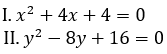### SBI PO Quantitative Aptitude Quiz

Here, we are providing free crash course for SBI PO as there is left not enough time to deal in details. The questions asked in the quantitative aptitude section are calculative and very time-consuming. But once dealt with proper strategy, speed, and accuracy, this section can get you the maximum marks in the examination. Following is the Quantitative Aptitude quiz to help you practice with the best of latest pattern questions

Q1. Two vessels contain a mixture of Spirit and water. In the first vessel the ratio of Spirt to water is 8 : 3 and in the second vessel the ratio is 5 : 1. A 35 litre cask is filled from these vessels so as to contain a mixture of Spirit and water in the ratio of 4 : 1. How many litres are taken from the first vessels ?
11 litres
22 litres
16.5 litres
27.5 litres
None of these

Q2. There are 2 bottles containing a mixture of wine, water and alcohol. The first bottle contains wine, water and alcohol in the ratio 3 : 5 : 2. The second bottle contains water and wine in the ratio 5 : 4. 1litre of the first and 2 litres of the second are mixed together. What fraction of the mixture is alcohol?
1/15 litres
6/13 litres
2/15 litres
6/19 litres
None of these

Q3. If 600 men dig a 5.5 m wide, 4 m deep and 405 m long canal in half an hour, then how long a canal will 2500 men working for 6 hrs, dig if it is 10 m wide and 8 m deep?
6452 m
5568 ¾ m
2694 ⅓ m
4082 m
None of these

Q4. The age of Sulekha and Arunima are in the ratio of 9 : 8 respectively. After 5 years the ratio of their age will be 10 : 9. What is the difference (in years) between their age?
4 years
5 years
6 years
7 years
9 years

Q5. A man crosses a stationary bus in 18 seconds. The same bus crosses a pole in 4 seconds. What is the respective ratio between the speed of the bus and the speed of the man?
9 : 2
9 : 4
18 : 5
Cannot be determined
7: 5

Q6. Train A travelling at 52 km/hr crosses another train B three fourth of train A’s length in 14 sec which is travelling in opposite direction at 38 km/hr. Train A passed a railway platform in 36 seconds. Find the length of platform.
200 m
300 m
320 m
400 m
450 m

Directions (7-10): Given below are 3 statements with each question, you have to decide that which of the following statement/statements are necessary to answer the question.

Q7. At what time will a train reach Lucknow from Patna?
A. The train crosses another train of equal length of 97.5 m and running in opposite direction in 9 sec.
B. The train leaves Patna at 11:15 am for Lucknow, which is at a distance of 567 km.
C. The 97.50-m-long train crosses a signal pole in 5 sec.

Only A
B and C together
A and C together
All statements are required
Only B

Q8. Find the height of an equilateral triangle.
A. Perimeter of the triangle is equal to the perimeter of the rectangle whose length and breadth are in the ratio of 5 :3.
B. Perimeter of a square is known, which is twice the perimeter of the triangle.
C. Area of the triangle is known.

Any two of them together.
Any one of them
Only C
Either B or C alone
A and either B or C

Q9. What is the value of a two–digit number?
A. The sum of the digits is 5.
B. The difference of the squares of the digits is 15.
C. The difference of their digits is 3.

A and B together are sufficient
B and C together are sufficient
C and A together are sufficient
Any one pair of A and B, B and C or C and A is sufficient

Q10. A boat takes 2 hours to travel from point A to B in still water. To find out its speed upstream, which of the following information is/are required?
A. Distance between point A and B.
B. Time taken to travel downstream from B to A.
C. Speed of the stream of water.

All are required
Any one pair of A and B, B and C or C and A is sufficient.
Only A and B
Only A and C
None of these

Directions (11-15): Two equations I and II are given below in each question. You have to solve these equations and give answer

Q11.if x < y
if x > y
if x ≤ y
if x ≥ y
if x = y or no relation can be established

Q12.if x < y
if x > y
if x ≤ y
if x ≥ y
if x = y or no relation can be established

Q13.if x < y
if x > y
if x ≤ y
if x ≥ y
if x = y or no relation can be established

Q14.if x < y
if x > y
if x ≤ y
if x ≥ y
if x = y or no relation can be established

Q15.if x < y
if x > y
if x ≤ y
if x ≥ y
if x = y or no relation can be established

You May also like to Read: